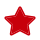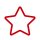Updated at: 2022-08-03 GMT+08:00

# PG_STATS

PG_STATS displays the single-column statistics stored in the pg_statistic table.

Table 1 PG_STATS columns

Name

Type

Reference

Description

schemaname

name

PG_NAMESPACE.nspname

Name of the schema that contains the table

tablename

name

PG_CLASS.relname

Name of the table

attname

name

PG_ATTRIBUTE.attname

Column name

inherited

boolean

-

Includes inherited sub-columns if the value is true; otherwise, indicates the column in a specified table.

null_frac

real

-

Percentage of column entries that are null

avg_width

integer

-

Average width in bytes of column's entries

n_distinct

real

-

• Estimated number of distinct values in the column if the value is greater than 0
• Negative of the number of distinct values divided by the number of rows if the value is less than 0

The negated form is used when ANALYZE believes that the number of distinct values is likely to increase as the table grows.

The positive form is used when the column seems to have a fixed number of possible values. For example, -1 indicates a unique column in which the number of distinct values is the same as the number of rows.

n_dndistinct

real

-

Number of unique non-null data values in the dn1 column

• Exact number of distinct values if the value is greater than 0
• Negative of the number of distinct values divided by the number of rows if the value is less than 0 (For example, if the value of a column appears twice in average, set n_dndistinct=-0.5.)
• The number of distinct values is unknown if the value is 0.

most_common_vals

anyarray

-

List of the most common values in a column. If this combination does not have the most common values, it will be NULL.

most_common_freqs

real[]

-

List of the frequencies of the most common values, that is, the number of occurrences of each value divided by the total number of rows. (NULL if most_common_vals is NULL)

histogram_bounds

anyarray

-

List of values that divide the column's values into groups of equal proportion. The values in most_common_vals, if present, are omitted from this histogram calculation. This field is null if the field data type does not have a < operator or if the most_common_vals list accounts for the entire population.

correlation

real

-

Statistical correlation between physical row ordering and logical ordering of the column values. It ranges from -1 to +1. When the value is near to -1 or +1, an index scan on the column is estimated to be cheaper than when it is near to zero, due to reduction of random access to the disk. This column is null if the column data type does not have a < operator.

most_common_elems

anyarray

-

Specifies a list of non-null element values most often appearing.

most_common_elem_freqs

real[]

-

Specifies a list of the frequencies of the most common element values.

elem_count_histogram

real[]

-

Specifies a histogram of the counts of distinct non-null element values.